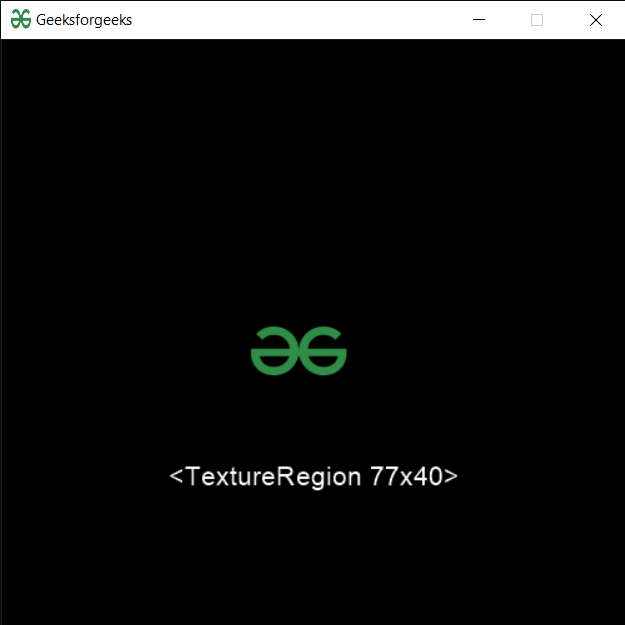In this article we will see how we can load a texture in PYGLET module in python. Pyglet is easy to use but powerful library for developing visually rich GUI applications like games, multimedia etc. A window is a “heavyweight” object occupying operating system resources. Windows may appear as floating regions or can be set to fill an entire screen (fullscreen). In order to load a file i.e resource we use resource module of pyglet. This module allows applications to specify a search path for resources. Relative paths are taken to be relative to the application’s __main__ module. Loaded texture of the named image will be loaded as a single OpenGL texture. If the dimensions of the image are not powers of 2 a TextureRegion will be returned.

We can create a window object with the help of command given below

```# creating a window
window = pyglet.window.Window(width, height, title)
```

In order to do this we use `texture` method with the pyglet.resource

Syntax : resource.texture(name)

Argument : It takes string i.e file name as argument

Return : It returns Texture object

Below is the implementation

 `# importing pyglet module  ` `import` `pyglet  ` `import` `pyglet.window.key as key ` `   `  `# width of window  ` `width ``=` `500` `   `  `# height of window  ` `height ``=` `500` `   `  `# caption i.e title of the window  ` `title ``=` `"Geeksforgeeks"` `   `  `# creating a window  ` `window ``=` `pyglet.window.Window(width, height, title)  ` `   `  `# text   ` `text ``=` `"Welcome to GeeksforGeeks"` `  `  `# creating label with following proeprties ` `# font = cooper ` `# position = 250, 150 ` `# anchor position = center ` `label ``=` `pyglet.text.Label(text,  ` `                          ``font_name ``=``'Cooper'``,  ` `                          ``font_size ``=` `16``,  ` `                          ``x ``=` `250``,   ` `                          ``y ``=` `150``,  ` `                          ``anchor_x ``=``'center'``,   ` `                          ``anchor_y ``=``'center'``) ` ` `  ` `  `# creating a batch  ` `batch ``=` `pyglet.graphics.Batch() ` ` `  `# loading geeksforgeeks image ` `image ``=` `pyglet.image.load(``'gfg.png'``) ` ` `  ` `  `# creating sprite object ` `# it is instance of an image displayed on-screen ` `sprite ``=` `pyglet.sprite.Sprite(image, x ``=` `200``, y ``=` `230``) ` `   `  `# on draw event  ` `@window``.event  ` `def` `on_draw():  ` `       `  `    ``# clear the window  ` `    ``window.clear()  ` `       `  `    ``# draw the label ` `    ``label.draw()  ` `     `  `    ``# draw the image on screen ` `    ``sprite.draw() ` `       `  `# key press event      ` `@window``.event  ` `def` `on_key_press(symbol, modifier):  ` `   `  `    ``# key "C" get press  ` `    ``if` `symbol ``=``=` `key.C:  ` `         `  `        ``# printng the message ` `        ``print``(``"Key : C is pressed"``) ` `         `  `# image for icon  ` `img ``=` `image ``=` `pyglet.resource.image(``"gfg.png"``)  ` ` `  `# setting image as icon  ` `window.set_icon(img)  ` ` `  ` `  `# loading a texture ` `value ``=` `pyglet.resource.texture(``"gfg.png"``) ` ` `  `# setting text  of label ` `label.text ``=` `str``(value) ` ` `  `# start running the application  ` `pyglet.app.run()  `

Output :Attention geek! Strengthen your foundations with the Python Programming Foundation Course and learn the basics.

To begin with, your interview preparations Enhance your Data Structures concepts with the Python DS Course.

My Personal Notes arrow_drop_upCheck out this Author's contributed articles.

If you like GeeksforGeeks and would like to contribute, you can also write an article using contribute.geeksforgeeks.org or mail your article to contribute@geeksforgeeks.org. See your article appearing on the GeeksforGeeks main page and help other Geeks.

Please Improve this article if you find anything incorrect by clicking on the "Improve Article" button below.

Article Tags :

Be the First to upvote.

Please write to us at contribute@geeksforgeeks.org to report any issue with the above content.